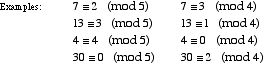index: click on a letter A B C D E F G H I J K L M N O P Q R S T U V W X Y Z A to Z index index: subject areas numbers & symbols sets, logic, proofs geometry algebra trigonometry advanced algebra & pre-calculus calculus advanced topics probability & statistics real world applications multimedia entrieswww.mathwords.com about mathwords website feedback

 Modular Equivalence Two integers are equivalent mod n if they leave the same remainder when divided by n. For example, 5 and 17 are equivalent mod 4 since they both have remainder 1 when divided by 4. We write 5 ≡ 17 (mod 4).See also